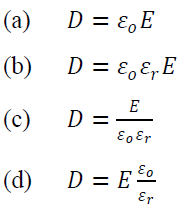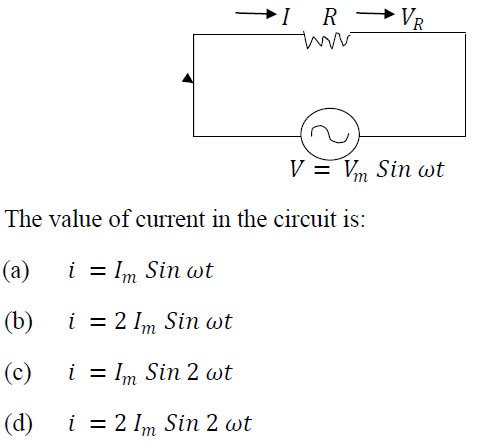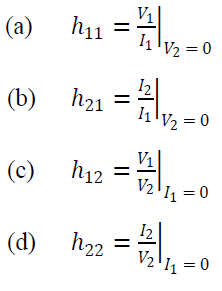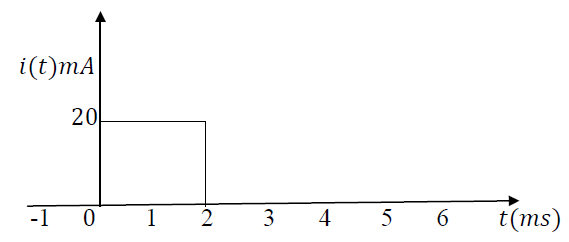### Solved Question Paper for Lecturer in Electrical Engineering in DTTE, GNCTD conducted by UPSC

11. For any medium, electric flux density D is related to electric field intensity E by the equation:12. In any linear resistive network, the voltage across or the current through any resistor or source may be calculated by adding algebraically all the individual voltages or currents caused by the separate independent sources acting alone, with all other independent voltage sources replaced by short circuits and all other independent current sources replaced by open circuits. Which one of the following theorems states the above definition?
(a) Maximum power transfer theorem
(b) Superposition theorem
(c) Maxwell’s theorem
(d) Source transformation theorem

13. Consider the following circuit:14. If two sinusoidal waves whose phases are to be compared:
1. Both be written as sine waves or both cosine waves
2. Both be written with positive amplitudes
3. Each has the same frequency
4. One wave should be sine and the other cosine wave

Which of the above statements are correct?
(a) 1, 2 and 4 only
(b) 1, 3 and 4 only
(c) 1, 2 and 3 only
(d) 2, 3 and 4 only

15. The drawback of 𝑚-derived filter is removed by connecting number of sections in addition to prototype and 𝑚-derived sections with terminating:
(a) Full sections
(b) One-fourth sections
(c) Half sections
(d) Square of three-fourth sections

16. The hybrid parameter of the open circuit reverse voltage gain is indicated as:17. Two identical 750 turn coils 𝐴 and 𝐵 lie in parallel planes. A current changing at the rate of 1500 𝐴/𝑠 in 𝐴 induces an emf of 11.25 𝑉 in 𝐵. If the self-inductance of each coil is 15 mH, then the percentage of the flux produced in coil 𝐴 which links the turns of 𝐵 will be:
(a) 40 %
(b) 50 %
(c) 60 %
(d) 70 %

18. What is the capacitor voltage that is associated with the current for the wave form shown when the value of the capacitance is 5 𝜇F?(a) 𝑣(𝑡)=1
(b) 𝑣(𝑡)=2
(c) 𝑣(𝑡)=4
(d) 𝑣(𝑡)=8

19. Current measured during a test is 30.4 𝐴, flowing in a resistor of 0.105 Ω . It is noted that the ammeter reading is low by 1.2 % and the marked resistance is high by 0.3 %. The true power will be nearly:
(a) 79.5 𝑊
(b) 89.3 𝑊
(c) 99.1 𝑊
(d) 109.0 𝑊

20. Schering Bridge is used for the measurement of:
(a) Capacitors
(b) Inductors
(c) Q-of inductors
(d) Medium value resistors

Share:

## Featured Post

### UPSC Civil Service Preliminary Paper-1 Previous Year Solved Question Papers

Civil Service Preliminary Paper-1 Previous Year Solved Questions for the year 2019 Civil Service Preliminary Paper-1 Previous Year Solved Qu...Name

Email *

Message *